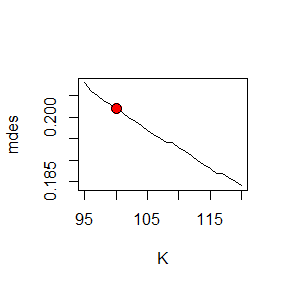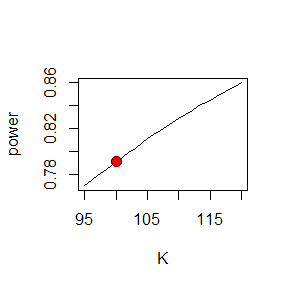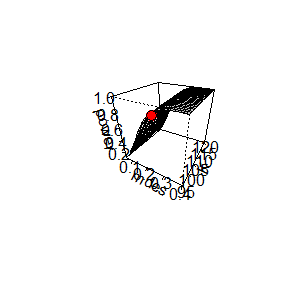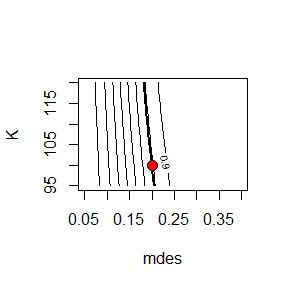# Problem

Institue of Education Science (IES) reported that evidence on effectiveness of Saxon Math curriclum on secondary school student’s algebra subject is lacking and further research is needed (U.S. Department of Education, 2016). To evaluate the effectiveness Saxon Math curriculum, assume a 3-level cluster randomized trial is considered where schools are randomly assigned to treatment (Saxon Math curriclum) and a control condition. In the grant proposal number of schools, teachers, and students, and the cost associated with data collection from this sample needs to be justified. We will use PowerUpR package (Bulus & Dong, 2016) an implementation of PowerUp! (Dong & Maynard, 2013) software in R environment (R Core Team, 2017).

## Statistical Model

Due to nesting of students within classrooms and nesting of classrooms within schools, the treatment effect ($$\delta$$) can be estimated via the following 3-level hierarchical linear model (HLM, Raudenbush & Bryk, 2002)

$\begin{eqnarray} L1: Y_{ijk} &=& \beta_{0jk} + \beta_{1jk}X + r_{ijk}, \quad r_{ijk} \thicksim N(0,\sigma_{|X}^2) \newline L2: \beta_{0jk} &=& \gamma_{00k} + \gamma_{01k}W + \mu_{0jk}, \quad \mu_{0jk} \thicksim N(0,\tau_{2|W}^2) \newline \beta_{1jk} &=& \gamma_{10k} \newline L3: \gamma_{00k} &=& \xi_{001} + \delta T_k + \xi_{002}V + \varsigma_{00k}, \quad \varsigma_{00k} \thicksim N(0,\tau_{3|V}^2) \newline \gamma_{01k} &=& \xi_{010} \newline \gamma_{10k} &=& \xi_{100} \end{eqnarray}$

where, $$Y$$: Student algebra posttest score. $$X$$: Student algebra pretest score. $$W$$: Classroom mean algebra pretest score. $$V$$: School mean algebra pretest score. $$T$$: Treatment status. Consider the following hypothetical values for design parameters

• Proportion of variance in algebra posttest at the school level ($$\tau_3^2$$): $$.15$$
• Proportion of variance in algebra posttest at the classroom level ($$\tau_2^2$$): $$.05$$
• Proportion of variance in algebra posttest at the student level ($$\sigma^2$$): $$.65$$
• Proportion of variance in algebra posttest explained by school mean algebra pretest: ($$R_3^2 = 1 - \frac{\tau_{3|V}^2}{\tau_{3}^2}$$): $$.45$$
• Proportion of variance in algebra posttest explained by classroom mean algebra pretest: ($$R_2^2 = 1 - \frac{\tau_{2|W}^2}{\tau_{2}^2}$$): $$.50$$
• Proportion of variance in algebra posttest explained by student algebra pretest: ($$R_1^2 = 1 - \frac{\sigma_{|X}^2}{\sigma^2}$$): $$.55$$

Then, intraclass correlation coefficients are

• $$\rho_2 = \tau_2^2 / (\sigma^2 + \tau_2^2 + \tau_3^2) = .05 /(.65 + .15 + .05) = 0.059$$
• $$\rho_3 = \tau_3^2 / (\sigma^2 + \tau_2^2 + \tau_3^2) = .15 /(.65 + .15 + .05) = 0.176$$

## Minimum Detectable Effect Size (MDES)

### Scenario 1: Find MDES

Further assumptions

• Alpha level ($$\alpha$$): $$0.05$$
• Number of schools ($$K$$): $$83$$
• Average number of classrooms per school ($$J$$): $$2$$
• Average number of students per classroom ($$n$$) : $$10$$
• Statistical power ($$1-\beta$$): $$.80$$

Given design parameters and further assumptions, what is minimum detectable effect size?

library(PowerUpR)
design1 <- mdes.cra3r3(power=.80, rho2=.06, rho3=.18,
g3=1, R12=.55, R22=.50, R32=.45,
P=.40, n=10, J=2, K=83)
print(design1$mdes) ## mdes 95% LCL 95% UCL ## [1,] 0.23 0.069 0.392 ## Statistical Power ### Scenario 2: Find Statistical Power Further assumptions • Alpha level ($$\alpha$$): $$0.05$$ • Number of schools ($$K$$): $$60$$ • Average number of classrooms per school ($$J$$): $$2$$ • Average number of students per classroom ($$n$$) : $$10$$ • Minimum detectable effect size (MDES): $$.232$$ Given design parameters and further assumptions, what is statistical power? design2 <- power.cra3r3(mdes=.23, rho2=.06, rho3=.18, g3=1, R12=.55, R22=.50, R32=.45, P=.40, n=10, J=2, K=83) print(design2$power)
##  0.8

## Minimum Required Sample Size (MRSS)

### Scenario 3: Number of Schools (K)

Further assumptions

• Alpha level ($$\alpha$$): $$0.05$$
• Average number of classrooms per school ($$J$$): $$2$$
• Average number of students per classroom ($$n$$) : $$10$$
• Statistical power ($$1-\beta$$): $$.80$$
• Minimum detectable effect size (MDES): $$.232$$

Given design parameters and further assumptions, how many schools should be recruited?

design3 <- mrss.cra3r3(power=.80, mdes=.23, rho2=.06, rho3=.18,
g3=1, R12=.55, R22=.50, R32=.45,
P=.40, n=10, J=2)
print(design3$round.mrss) ## n J K mdes power ## [1,] 10 2 83 0.23 0.8 ### Scenario 4: Number of Classrooms (J) Further assumptions • Alpha level ($$\alpha$$): $$0.05$$ • Number of schools ($$K$$): $$60$$ • Average number of students per classroom ($$n$$) : $$10$$ • Statistical power ($$1-\beta$$): $$.80$$ • Minimum detectable effect size (MDES): $$.232$$ Given design parameters and further assumptions, how many classrooms should be recruited per school on average? design4 <- mrss.cra3r3(power=.80, mdes=.23, rho2=.06, rho3=.18, g3=1, R12=.55, R22=.50, R32=.45, P=.40, n=10, K=83) print(design4$round.mrss)
##       n J  K mdes power
## [1,] 10 2 83 0.23   0.8

### Scenario 5: Number of Students (n)

Further assumptions

• Alpha level ($$\alpha$$): $$0.05$$
• Number of schools ($$K$$): $$60$$
• Average number of classrooms per school ($$J$$): $$2$$
• Statistical power ($$1-\beta$$): $$.80$$
• Minimum detectable effect size (MDES): $$.232$$

Given design parameters and further assumptions, how many studetns should be recruited per classroom on average?

design5 <- mrss.cra3r3(power=.80, mdes=.23, rho2=.06, rho3=.18,
g3=1, R12=.55, R22=.50, R32=.45,
P=.40, J=2, K=83)
print(design5$round.mrss) ## n J K mdes power ## [1,] 10 2 83 0.23 0.8 ## Optimal Sample ### Scenario 6: Constrain Power Further assumptions • Alpha level ($$\alpha$$): $$0.05$$ • Statistical power ($$1-\beta$$): $$.80$$ • Minimum detectable effect size (MDES): $$.232$$ • Marginal cost per student ($$cn$$): $$10$$ • Marginal cost per classroom ($$cJ$$): $$200$$ • Marginal cost per school ($$cK$$): $$500$$ Given design parameters and further assumptions, how many schools, classrooms and students should be recruited to achieve 80% power while minimizing the total cost? What are alternative combinations of $$n$$, $$J$$, and $$K$$? design6 <- optimal.cra3r3(cn=10, cJ=200, cK=500, constrain="power", power=.80, gm=5, ncase=20, mdes=.23, rho2=.06, rho3=.18, g3=1, R12=.55, R22=.50, R32=.45, P=.40) print(design6$round.optim)
##       n J  K  cost  mdes power
## [1,] 13 1 97 80510 0.231 0.796
print(design6$integer.optim) ## n J K cost mdes power ## [1,] 13 1 98 81340 0.230 0.800 ## [2,] 1 7 96 189120 0.230 0.800 ## [3,] 11 1 101 81810 0.230 0.800 ## [4,] 16 1 95 81700 0.230 0.800 ## [5,] 1 8 92 200560 0.230 0.800 ## [6,] 4 2 99 97020 0.230 0.800 ## [7,] 19 1 93 82770 0.230 0.801 ## [8,] 3 3 93 110670 0.230 0.801 ## [9,] 14 1 97 81480 0.230 0.801 ## [10,] 17 1 94 81780 0.230 0.799 ## [11,] 15 1 96 81600 0.230 0.801 ## [12,] 21 1 92 83720 0.230 0.801 ## [13,] 2 4 94 129720 0.230 0.799 ## [14,] 20 1 92 82800 0.230 0.799 ## [15,] 12 1 99 81180 0.230 0.799 ## [16,] 5 2 94 94000 0.230 0.802 ## [17,] 1 6 102 179520 0.229 0.802 ## [18,] 1 6 101 177760 0.231 0.798 ## [19,] 18 1 93 81840 0.231 0.798 ## [20,] 18 1 94 82720 0.229 0.802 Note that MDES values in the output are calculated at the specified power value (.80), and power values in the output are calculated at the specified MDES value (.23). ### Scenario 7: Constrain Power and Number of Classrooms Further assumptions • Alpha level ($$\alpha$$): $$0.05$$ • Statistical power ($$1-\beta$$): $$.80$$ • Minimum detectable effect size (MDES): $$.232$$ • Average number of classrooms per school ($$J$$): $$2$$ • Marginal cost per student ($$cn$$): $$10$$ • Marginal cost per classroom ($$cJ$$): $$200$$ • Marginal cost per school ($$cK$$): $$500$$ Given design parameters and further assumptions, how many schools, and students should be recrutied to achieve 80% power while minimizing the total cost? design7 <- optimal.cra3r3(cn=10, cJ=200, cK=500, constrain="power", power=.80, J=2, mdes=.23, rho2=.06, rho3=.18, g3=1, R12=.55, R22=.50, R32=.45, P=.40) print(design7$round.optim)
##      n J  K  cost  mdes power
## [1,] 8 2 85 90100 0.231 0.797
print(design7$integer.optim) ## n J K cost mdes power ## [1,] 4 3 87 106140 0.230 0.800 ## [2,] 10 2 83 91300 0.230 0.800 ## [3,] 2 6 84 152880 0.230 0.800 ## [4,] 9 2 84 90720 0.230 0.799 ## [5,] 8 2 86 91160 0.230 0.802 ## [6,] 3 4 85 120700 0.231 0.798 ## [7,] 5 3 83 103750 0.231 0.798 ## [8,] 5 3 84 105000 0.229 0.803 ## [9,] 3 4 86 122120 0.229 0.803 ## [10,] 7 2 87 90480 0.231 0.797 Note that MDES values in the output are calculated at the specified power value (.80), and power values in the output are calculated at the specified MDES value (.23). ### Scenario 8: Constrain MDES and Number of Classrooms Further assumptions • Alpha level ($$\alpha$$): $$0.05$$ • Statistical power ($$1-\beta$$): $$.80$$ • Minimum detectable effect size (MDES): $$.20$$ • Average number of classrooms per school ($$J$$): $$2$$ • Marginal cost per student ($$cn$$): $$10$$ • Marginal cost per classroom ($$cJ$$): $$200$$ • Marginal cost per school ($$cK$$): $$500$$ Given design parameters and further assumptions, how many schools, and students should be recruited to achieve an MDES of .20 while minimizing the total cost? design8 <- optimal.cra3r3(cn=10, cJ=200, cK=500, constrain="mdes", mdes=.20, J=2, power=.80, rho2=.06, rho3=.18, g3=1, R12=.55, R22=.50, R32=.45, P=.40) print(design8$round.optim)
##      n J   K   cost  mdes power
## [1,] 9 2 107 115560 0.204 0.786
print(design8$integer.optim) ## n J K cost mdes power ## [1,] 13 2 106 122960 0.2 0.800 ## [2,] 11 2 108 120960 0.2 0.800 ## [3,] 12 2 107 121980 0.2 0.801 ## [4,] 10 2 109 119900 0.2 0.799 ## [5,] 14 2 105 123900 0.2 0.799 ## [6,] 6 3 107 136960 0.2 0.801 ## [7,] 4 4 107 156220 0.2 0.802 ## [8,] 15 2 105 126000 0.2 0.802 ## [9,] 3 5 106 174900 0.2 0.798 ## [10,] 3 5 107 176550 0.2 0.802 Note that MDES values in the output are calculated at the specified power value (.80), and power values in the output are calculated at the specified MDES value (.20). ### Scenario 9: Constrain Cost and Number of Classrooms Further assumptions • Alpha level ($$\alpha$$): $$0.05$$ • Statistical power ($$1-\beta$$): $$.80$$ • Minimum detectable effect size (MDES): $$.20$$ • Average number of classrooms per school ($$J$$): $$2$$ • Marginal cost per student ($$cn$$): $$10$$ • Marginal cost per classroom ($$cJ$$): $$200$$ • Marginal cost per school ($$cK$$): $$500$$ • Budget: $$130,000$$ Given design parameters and further assumptions, how many schools, and students should be recruited while maximizing the power? design9 <- optimal.cra3r3(cn=10, cJ=200, cK=500, constrain="cost", cost=130000, J=2, power=.80, mdes=.20, rho2=.06, rho3=.18, g3=1, R12=.55, R22=.50, R32=.45, P=.40) print(design9$round.optim)
##       n J  K   cost  mdes power
## [1,] 59 2 62 128960 0.252 0.602
print(design9$integer.optim) ## n J K cost mdes power ## [1,] 60 2 62 130200 0.252 0.603 ## [2,] 58 2 63 129780 0.250 0.609 ## [3,] 63 2 60 129600 0.257 0.589 ## [4,] 62 2 61 130540 0.254 0.596 ## [5,] 57 2 64 130560 0.248 0.616 ## [6,] 61 2 61 129320 0.255 0.596 ## [7,] 56 2 64 129280 0.249 0.616 ## [8,] 64 2 60 130800 0.257 0.589 ## [9,] 59 2 62 128960 0.252 0.602 ## [10,] 59 2 63 131040 0.250 0.610 Note that MDES values in the output are calculated at the specified power value (.80), and power values in the output are calculated at the specified MDES value (.20). ### Scenario 10: Constrain Cost, Number of Classrooms, and Number of Students Further assumptions • Alpha level ($$\alpha$$): $$0.05$$ • Statistical power ($$1-\beta$$): $$.80$$ • Minimum detectable effect size (MDES): $$.20$$ • Average number of students per classroom ($$J$$): $$20$$ • Average number of classrooms per school ($$J$$): $$2$$ • Marginal cost per student ($$cn$$): $$10$$ • Marginal cost per classroom ($$cJ$$): $$200$$ • Marginal cost per school ($$cK$$): $$500$$ • Budget: $$130,000$$ Given design parameters and further assumptions, how many schools should be recruited while maximizing the power? design10 <- optimal.cra3r3(cn=10, cJ=200, cK=500, constrain="cost", cost=130000, n=20, J=2, power=.80, mdes=.20, rho2=.06, rho3=.18, g3=1, R12=.55, R22=.50, R32=.45, P=.40) print(design10$round.optim)
##       n J   K   cost  mdes power
## [1,] 20 2 100 130000 0.202 0.791
print(design10$integer.optim) ## n J K cost mdes power ## [1,] 20 2 100 130000 0.202 0.791 ## [2,] 19 2 102 130560 0.201 0.798 ## [3,] 21 2 98 129360 0.204 0.784 ## [4,] 21 2 99 130680 0.203 0.789 ## [5,] 19 2 101 129280 0.202 0.794 ## [6,] 20 2 99 128700 0.203 0.787 ## [7,] 20 2 101 131300 0.201 0.795 ## [8,] 22 2 98 131320 0.204 0.786 ## [9,] 18 2 102 128520 0.201 0.796 ## [10,] 19 2 100 128000 0.203 0.790 Note that MDES values in the output are calculated at the specified power value (.80), and power values in the output are calculated at the specified MDES value (.20). ## Plots Users can produce 2D plots, 3D or contour plots by specifying pars and type arguments in plot function (S3 method for class ‘pars’). By default object returned from mdes functions produces plots where MDES is on top axis. Similarly, object returned from power functions produces plots where power is on top axis. For objects returned from mrss or optimal functions by default MDES is on top axis. Users can switch the order in pars statement for an alternative plot. type="p" corresponds to perspective plot and type="c" corresponds to contour plot. Note: First argument in pars statement should be either power or mdes. ### Plot X-Y Relationships ## MDES - MRSS(K) ## Red point indicates the design location plot(design10, pars=c("mdes","K"))## Power - MRSS(K) ## Red point indicates the design location plot(design10, pars=c("power","K"))### Plot X-Y-Z Relationships ## Perspective plot ## Red point indicates the design location plot(design10, type="p")## Contour plot ## Red point indicates the design location plot(design10, type="c")## Summary ## confidence intervals for MDES design11 <- optimal.to.mdes(design10) print(design11$mdes)
##       mdes 95% LCL 95% UCL
## [1,] 0.202    0.06   0.344
## percentile equivalance
mdes.to.pctl(design11)
##      pctl  95% LCL 95% UCL
## [1,] "58%" "52%"   "63%"

A 3-level hierarchical linear model is proposed to estimate treatment effect, where schools are randomly assigned to treatment and control conditions with 40% probability. We assume that proportion of variance in algebra posttest explained by school mean algebra pretest is 45%, proportion of variance in algebra posttest explained by classroom mean algebra pretest is 50%, proportion of variance in algebra posttest explained by student algebra pretest is 55%. Furthermore we assume intraclass correlation coefficient at the school level is 17.6%, and intraclass correlation coefficient at the classroom level is 5.9%.

Having $10 marginal cost per student,$200 marginal cost per classroom, $500 marginal cost per school, based on PowerUpR package (Bulus & Dong, 2017), with a budget of$130000 we can afford recruting 100 schools in total, each with two classrooms and 40 students on average. For a two-tailed hypothesis test for treatment effect, and Type I error rate of 5%, such sample is estimated to have statistical power of 79% for an effect size of .200, or estimated to detect an effect size as small as .202 95% CI[.060, .344] with statistical power of 80%, which is equaivalent to increasing an average student’s algebra posttest score from 50th percentile to 58th percentile.